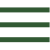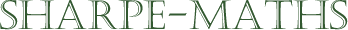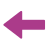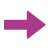Previous topic:Current topic:
Converting percentagesNext topic:
Finding a percentage of a number

### Percentage

Percentages are hard. Even though they are a concept that most people think they understand, there are many different types of problem, which on a surface level, appear to be similar, but actually require a wholly different method. In the section, I will be going through all of the important aspects of working with percentages, starting with what a percentage is and going on to look at reverse percentages and compound interest. Below is a list of sub-topics which we will work with here.

1. What is a percentage?
2. Converting between a percentage and other similar forms.
3. Finding a percentage of a quantity.
4. Increasing a quantity by a percentage
5. Decreasing a quantity by a percentage
6. Expressing one quantity as a percentage of another
7. Repeated increases and decreases over time
8. Undoing a percentage increase or decrease
9. Summary of question types